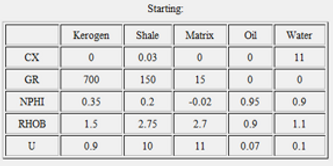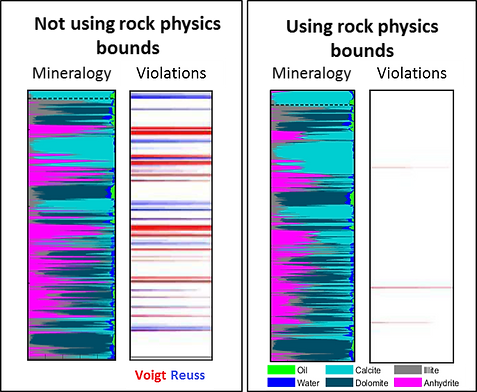top of page

## What is iMineralysis™ ?

iMineralysis™ is a fast, nonlinear petrophysical modeling and optimization tool based on artificial intelligence (genetic algorithm) designed to estimate lithology and fluid compositions in the most complex geological settings of rock and fluid properties. It generates a multimineral solution that closely predicts the input logs and outputs the fractions of fluids and minerals present in the rock. It is the most advanced multimineral solver in the market.

iMineralysis™ allows the user to define the initial set of coefficients and a range of a suspected uncertainty for each. Then, iMineralysis™ not only performs the estimation of mineral fractions, but also intelligently tests hundreds of combinations of coefficients within the specified ranges. Basically, it performs the multimineral analysis for thousands of possible cases in seconds! The final result is not only the mineral composition and modeled curves, but also the optimized matrix coefficients.

The software was originally developed by iReservoir in 2006. For the next 13 years, new features were added after being tested and used internally to provide services in many geologic environments. The first commercial version of the software was released in 2019.

No other commercially available multimineral inversion application offers the capabilities listed below.

Unique features of iMineralysis™

• Accelerates the connection from log data to results by testing thousands of models.

• Allows the estimation of the mineral coefficients (end-points) when performing multimineral analysis in areas where the coefficients are uncertain. Uses a genetic algorithm to optimize the coefficients within a given range of values for each one.

• Automates the time-consuming, manual trial-and-error process of estimating mineral constants.

• The increased speed allows access to new insights such as processing large data sets for rock composition, estimation of mineral constants in complex lithologies, and mapping of mineral properties (e.g., water resistivity, kerogen maturity, and clay composition)

• Optimizations can be performed for individual wells or groups of wells, allowing the generation of regional maps of mineral end points and errors.

• Provides estimates of of solutions to help the user determine which ones need better constraints.

• Incorporates elastic rock physics bounds to constrain the multimineral solution.

Example of the coefficient matrix showing the starting coefficient for the optimization process and the allowed ranges for each coefficient. Aggregate of minerals lumped into a single "Matrix" constituent can also be tested.

MatrixDifferent coefficients tested by the genetic algorithm (red) and the best solution for each iteration (blue). The initial coefficient is indicated in green. Horizontal axis is the iteration number and the vertical is the range of possible coefficients. By examining the convergence of the algorithm, the user can modify the input ranges to best honor the input data. Notice how the initial coefficient (green) is not necessarily equal to the optimal. Coefficients whose values are certain don't change during the optimization process.

CoefficientsTestMultimineral solution performed using the optimized coefficients. Mineral solution is shown on the left, and raw data (red) vs. predicted logs (blue) is shown on the right. Different models for different geologic intervals with different compositions can be optimized simultaneously. Logs can be usually modeled very closely because the optimization aims to minimize the distance between them and measured logs.

ResultsMatrix of coefficients for the different constituents before (left) and after (right) optimization.Errors

When iMineralysis is applied to individual wells in a large area, we can use the results to map spatial variations in mineral coefficients (end-points) as well as model errors. No other multimineral application in the market has this capability.Analysis of the model fitness can help to assess their non-uniqueness. Each dot represents a model tested in the optimization, colored by fitness from poor (red) to good (green). Horizontal axis in the distance between the best solution and other models; vertical axis the fitness of each model. iMineralysis allows the assessment of non-uniqueness and instabilities in the solution.

RockPhysicsBoundsIf the multimineral solution does not violate the elastic Voigt or Reuss bounds, it means the solution is plausible. If the bounds are violated, it means that there is a problem with the solution that requires further examination. iMineralysis can use these theoretical bounds to constrain the linear solver as well as the nonlinear optimization of matrix coefficients.

bottom of page